Courses
Courses for Kids
Free study material
Offline Centres
More

# NCERT Solutions for Class 6 Maths Chapter 13 - ExerciseLast updated date: 06th Dec 2023
Total views: 528.9k
Views today: 9.28k

## NCERT Solutions for Class 6 Maths Chapter 13 Symmetry (Ex 13.3) Exercise 13.3

Free PDF download of NCERT Solutions for Class 6 Maths Chapter 13 Exercise 13.3 (Ex 13.3) and all chapter exercises at one place prepared by expert teacher as per NCERT (CBSE) books guidelines. Class 6 Maths Chapter 13 Symmetry Exercise 13.3 Questions with Solutions to help you to revise complete Syllabus and Score More marks. Register and get all exercise solutions in your emails.

## Access NCERT Solutions for Maths Class 6 Chapter 13- Symmetry

Exercise 13.3

1. Find the number of lines of symmetry in each of the following shapes. How will you check your answer?

A)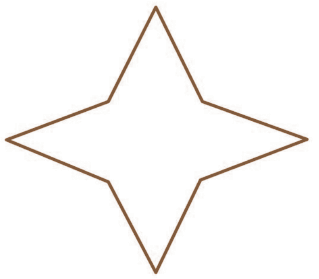Ans: We can see that the image can be divided identically in horizontal, vertical, and in a cross. So the line of symmetry of the image is 4.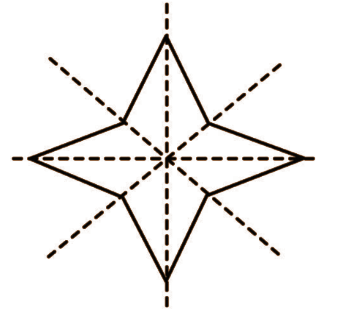B)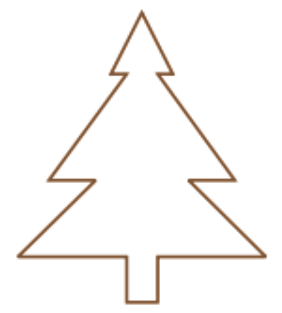Ans: We can divide the image vertically and no other way the image can be divided identically. So the line of symmetry of the image is 1.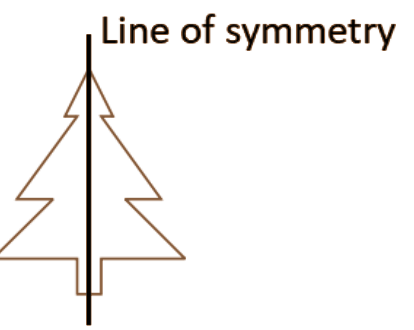C)Ans: The only way this image can be divided identically is a cross. So the line of symmetry of the image is 2.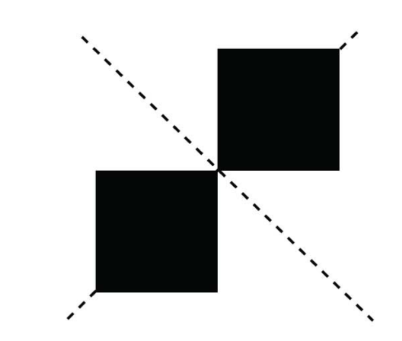D)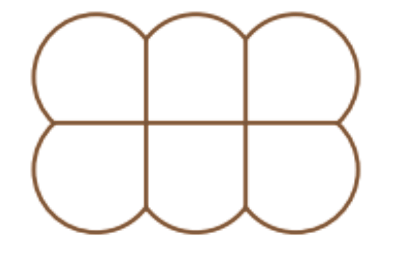Ans:  The given image can be divided identically in a horizontal and vertical way. So the line of symmetry of the image is 2.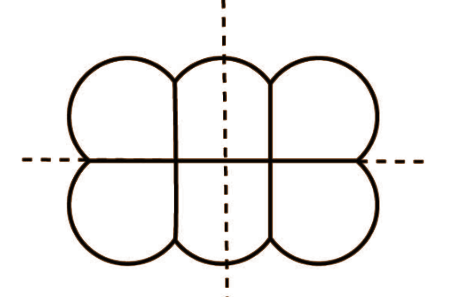E)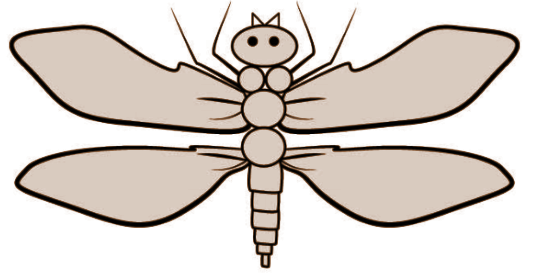Ans: The given image can be divided vertically to be identical. So the line of symmetry of the image is 1.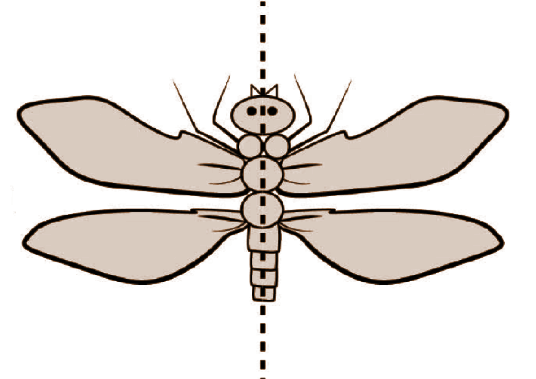F)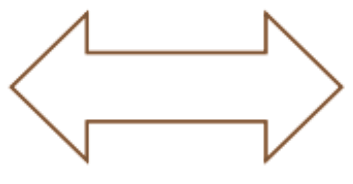Ans: This image can be divided symmetrically both in horizontal and vertical.  So the line of symmetry of the image is 2.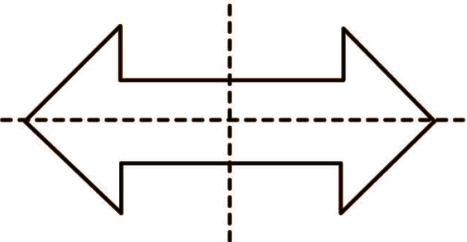2. Copy the following drawing on squared paper. Complete each one of them such that the resulting figure has two dotted lines as two lines of symmetry. How did you go about completing the picture?

A)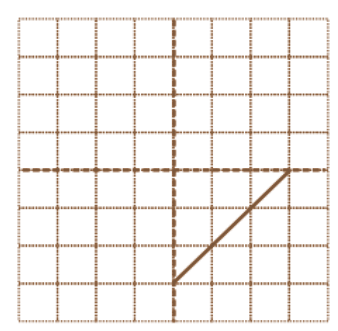Ans: The drawing is copied and drawn wisely such that the two dotted lines are now the line of symmetry of the drawing.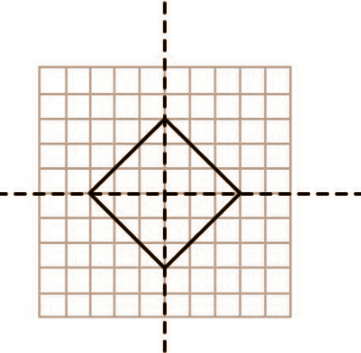B)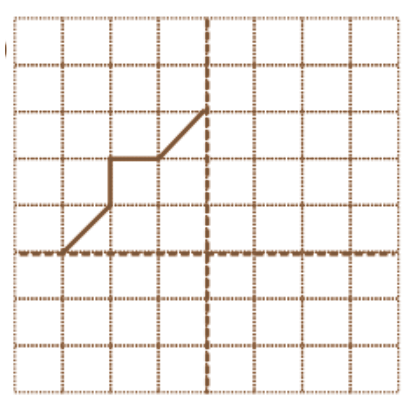Ans: The drawing is copied and drawn wisely such that the two dotted lines are now the line of symmetry of the drawing.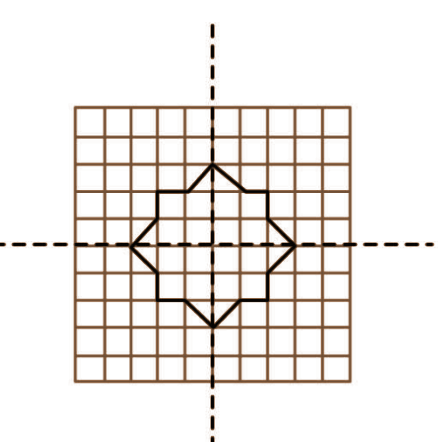C)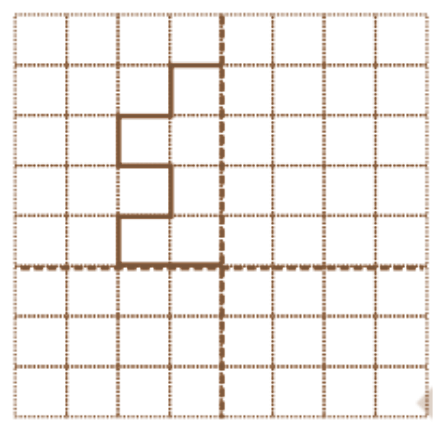Ans: The drawing is copied and drawn wisely such that the two dotted lines are now the line of symmetry of the drawing.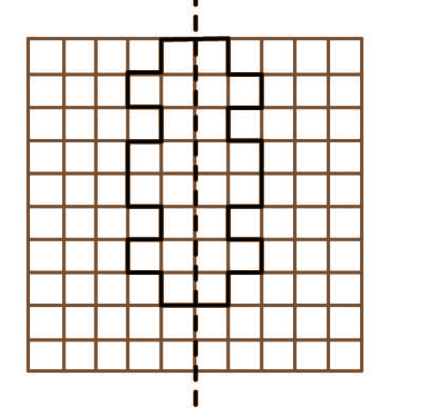D)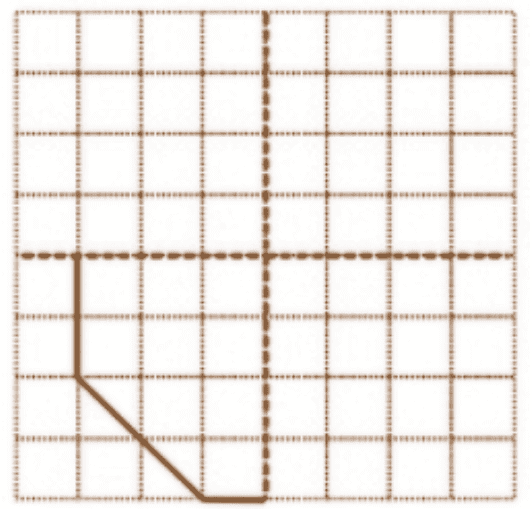Ans: The drawing is copied and drawn wisely such that the two dotted lines is now the line of symmetry of the drawing.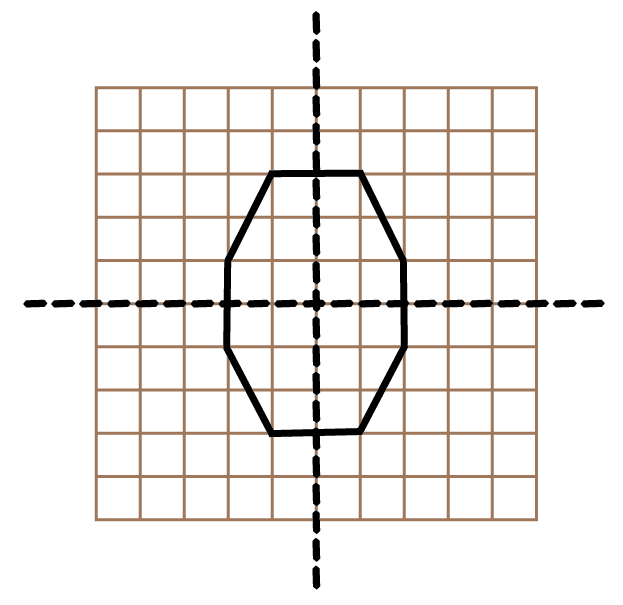E)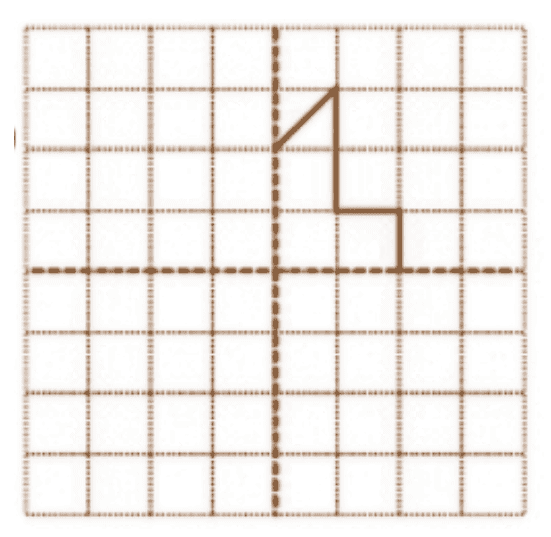Ans: The drawing is copied and drawn wisely such that the two dotted lines is now the line of symmetry of the drawing.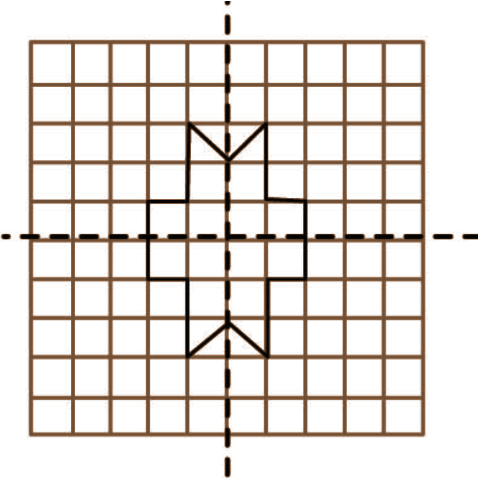F)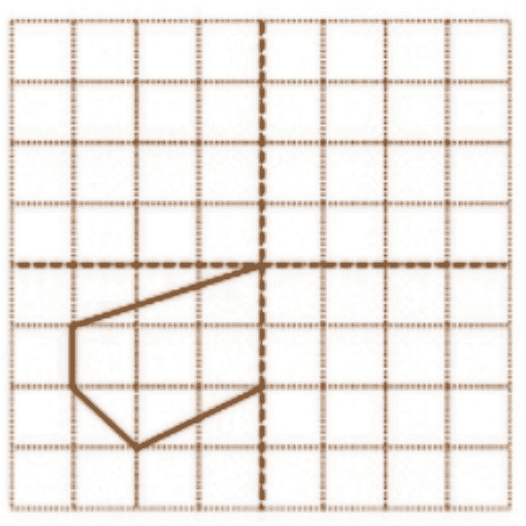Ans: The drawing is copied and drawn wisely such that the two dotted lines is now the line of symmetry of the drawing.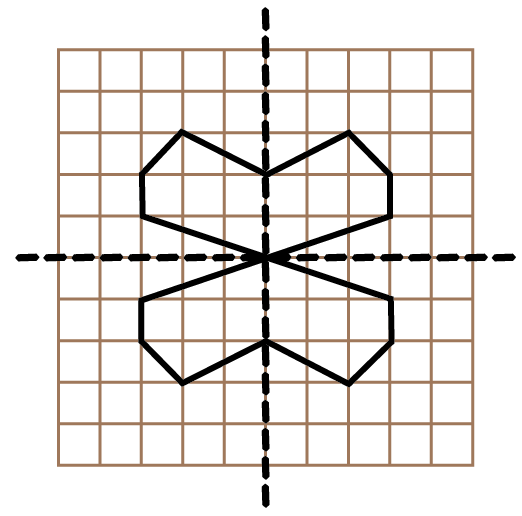3. In each figure below, a letter of the alphabet is shown along with a vertical line. Take the mirror image of the letter in the given line. Find which letters look the same after reflection (i.e., which letters look the same in the image) and which do not. Can you guess why?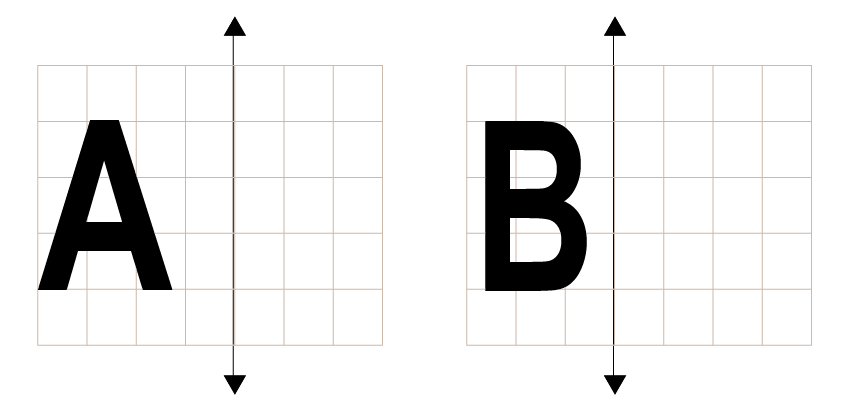Try for O, E, M, N, P, H, L, T, S, V, X.

Solution :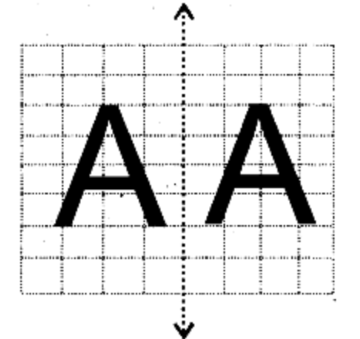The letter A looks similar after reflection because it is Symmetric.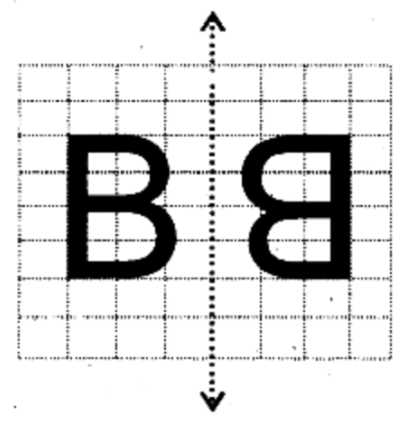The letter B doesn’t look the same after reflection because it is Asymmetric.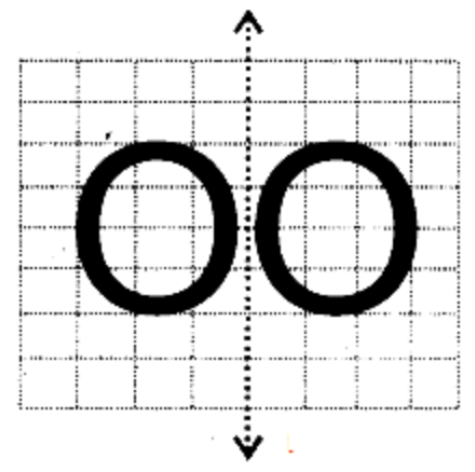The letter O looks similar after reflection because it is Symmetric.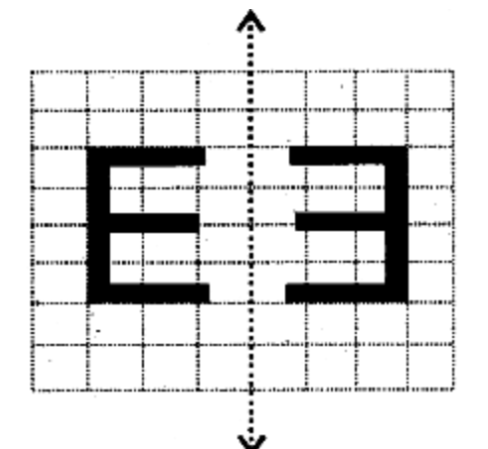The letter E doesn’t look the same after reflection because it is Asymmetric.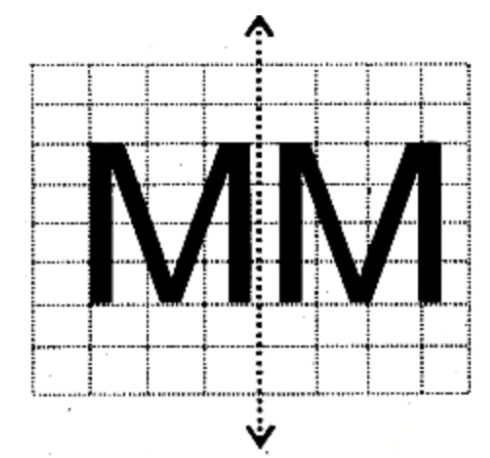The letter M looks the same after the reflection because it is Symmetric.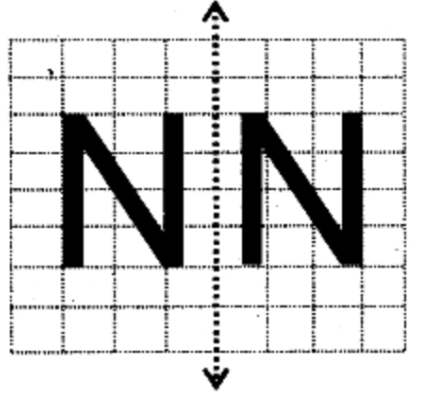The letter N doesn’t look the same after reflection because it is Asymmetric.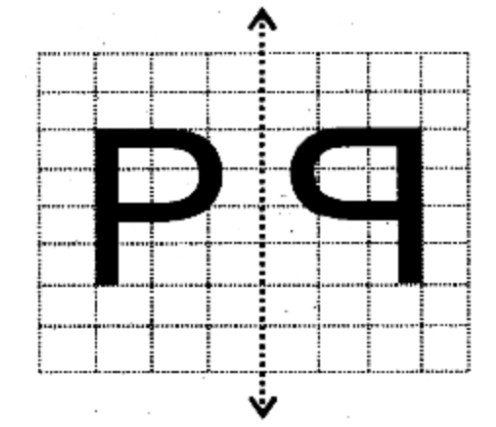The letter P doesn’t look the same after reflection because it is Asymmetric.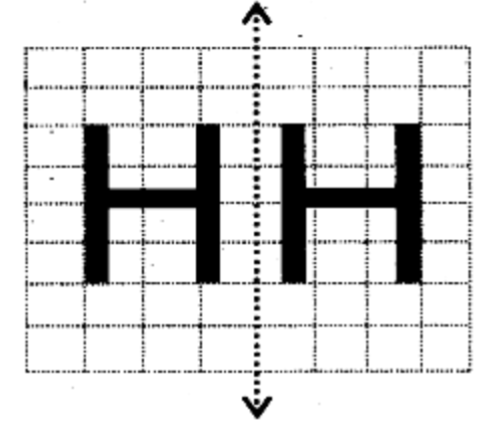The Letter H looks similar after the reflection because it is Symmetric.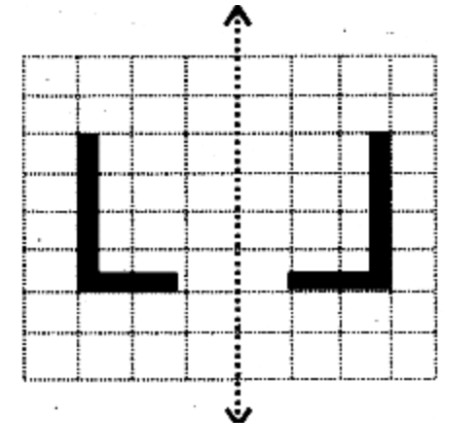The letter L doesn’t look the same after reflection because it is Asymmetric.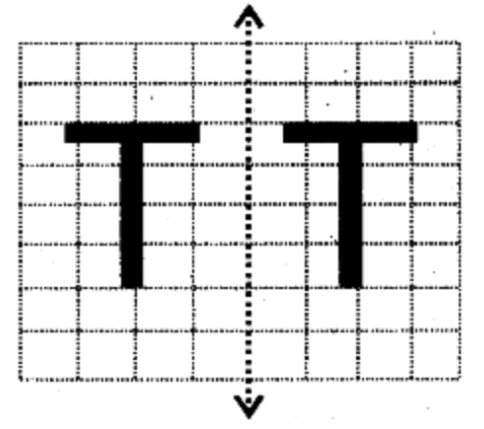The letter T looks similar after the reflection because it is Symmetric.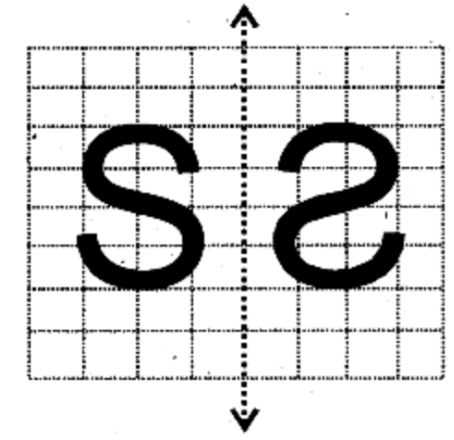The letter S doesn’t look the same after the reflection because it is Asymmetric.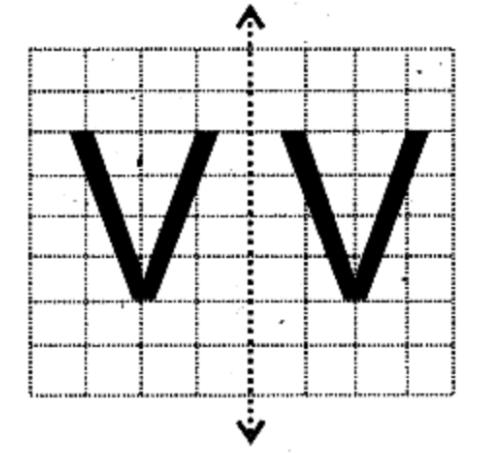The letter V looks the same after the reflection because it is Symmetric.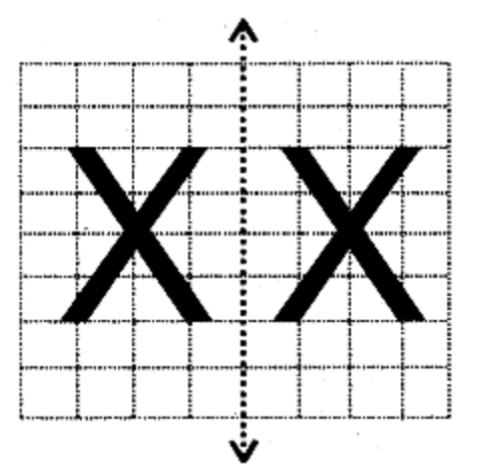The letter X looks the same after the reflection because it is Symmetric.

The letters that look the same after the reflection are H, M, O, T, V, X.

The letters that do not look the same after the reflection are E, L, N, P, S.

## NCERT Solutions for Class 6 Maths Chapter 13 Symmetry Exercise 13.3

Opting for the NCERT solutions for Ex 13.3 Class 6 Maths is considered as the best option for the CBSE students when it comes to exam preparation. This chapter consists of many exercises. Out of which we have provided the Exercise 13.3 Class 6 Maths NCERT solutions on this page in PDF format. You can download this solution as per your convenience or you can study it directly from our website/ app online.

Vedantu in-house subject matter experts have solved the problems/ questions from the exercise with the utmost care and by following all the guidelines by CBSE. Class 6 students who are thorough with all the concepts from the Subject Maths textbook and quite well-versed with all the problems from the exercises given in it, then any student can easily score the highest possible marks in the final exam. With the help of this Class 6 Maths Chapter 13 Exercise 13.3 solutions, students can easily understand the pattern of questions that can be asked in the exam from this chapter and also learn the marks weightage of the chapter. So that they can prepare themselves accordingly for the final exam.

Besides these NCERT solutions for Class 6 Maths Chapter 13 Exercise 13.3, there are plenty of exercises in this chapter which contain innumerable questions as well. All these questions are solved/answered by our in-house subject experts as mentioned earlier. Hence all of these are bound to be of superior quality and anyone can refer to these during the time of exam preparation. In order to score the best possible marks in the class, it is really important to understand all the concepts of the textbooks and solve the problems from the exercises given next to it.

Do not delay any more. Download the NCERT solutions for Class 6 Maths Chapter 13 Exercise 13.3 from Vedantu website now for better exam preparation. If you have the Vedantu app in your phone, you can download the same through the app as well. The best part of these solutions is these can be accessed both online and offline as well.